Get instant live expert help with Excel or Google Sheets“My Excelchat expert helped me in less than 20 minutes, saving me what would have been 5 hours of work!”

#### Post your problem and you’ll get Expert help in seconds.

Your message must be at least 40 characters
Our professional Expert are available now. Your privacy is guaranteed.

# How to Use the COUNTIF Function

We can use the excel COUNTIF Function to count cells in a range that meet a criteria. We can count cells with dates, numbers, and text that match the specified criteria. This function supports logical operators like >, <, <>, = but not wildcards like * and ? where partial matching is required. This steps below will walk through the process.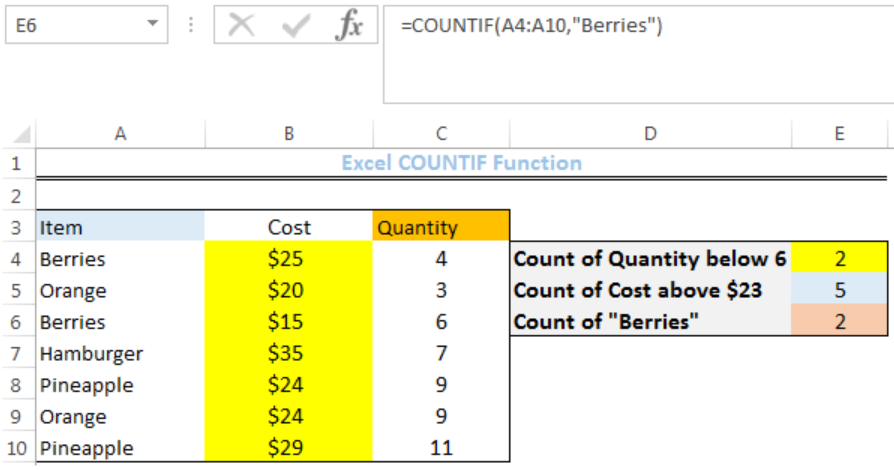Figure 1: How to Use the COUNTIF Function

## Syntax

`=COUNTIF(range,criteria)`

• Range is the range of values where we want a count
• Criteria is the VALUE which the COUNTIF FUNCTION returns a count for.

## Formula

• Count of quantity below 6: `=COUNTIF(C4:C10,"<6")`
• Count of cost above \$23: `=COUNTIF(B4:B10,">\$23")`
• Count of Berries: `=COUNTIF(A4:A10,"Berries")`

## Setting up the Data

We have items that have been bought, including the cost for each and the quantity. We will count the quantity below 6, cost above \$23, and the number of times berries appear.

• We will input the ITEMS in Column A
• We will input the COST in Column B
• We will input the QUANTITY in Column C
• COLUMN E is where we want the COUNTIF FUNCTION to return the results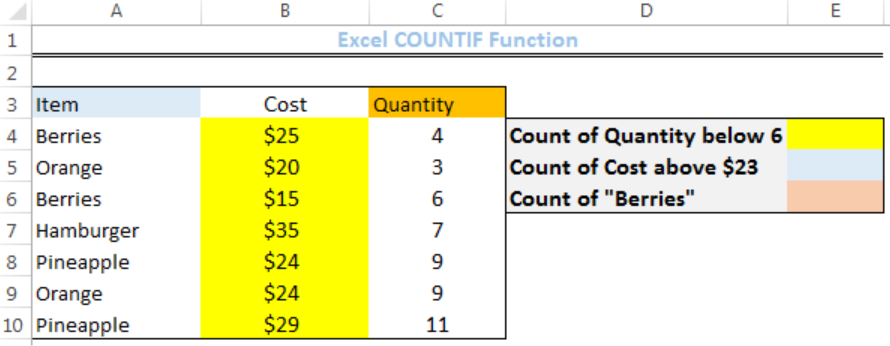Figure 2: Setting up the Data

## Count of Quantity Below 6

• We will click on Cell E4
• We will insert the formula below into the cell
`=COUNTIF(C4:C10,"<6")`
• We will press the enter key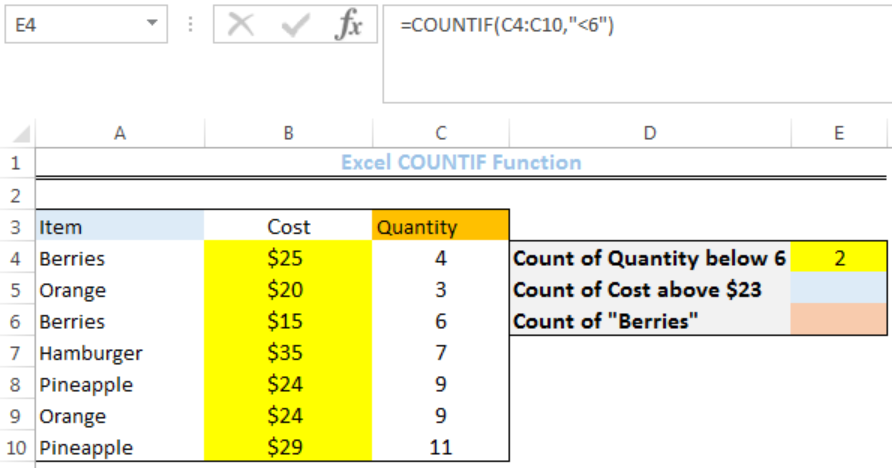Figure 3: Count of Quantity Below 6

## Count of Cost above \$23

• We will click on Cell E5
• We will insert the formula below into the cell
`=COUNTIF(B4:B10,">\$23")`
• We will press the enter key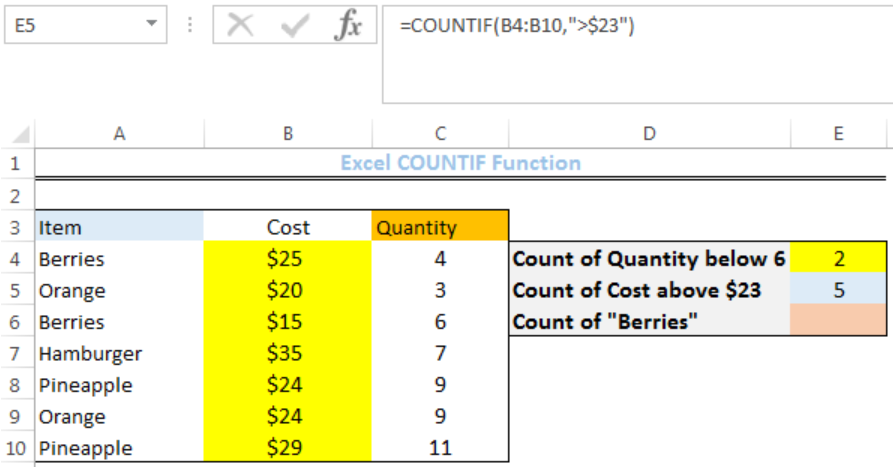Figure 4: Count of Cost above \$23

## Count of Berries

• We will click on Cell E6
• We will insert the formula below into the cell
`=COUNTIF(A4:A10,"Berries")`
• We will press the enter key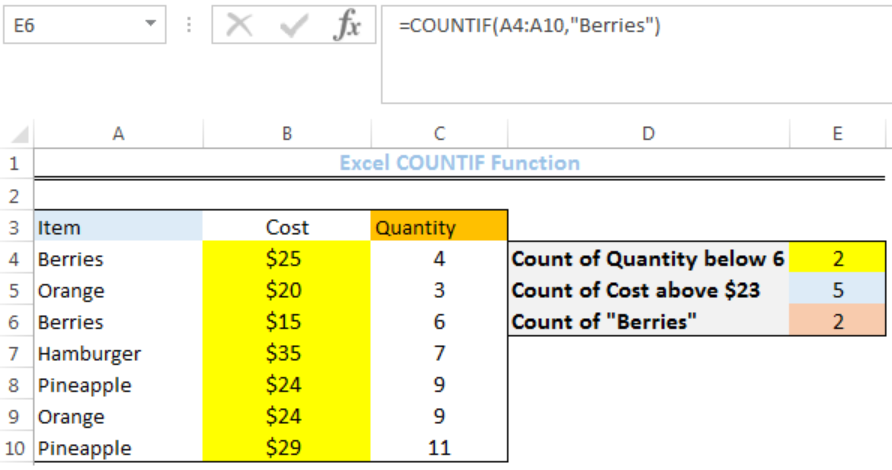Figure 5: Count of Berries

## Notes

• Wildcard characters such as ‘?’ and ‘*’ can also be used as a criteria. In this case, a question mark is used to match any single character while an asterisk is used to match any sequence of characters within cells.
• To find a question mark or asterisk with a literal meaning, use a tilde ‘~’ in before the question mark or asterisk (example ~? or ~*).

## Instant Connection to an Expert through our Excelchat Service

Most of the time, the problem you will need to solve will be more complex than a simple application of a formula or function. If you want to save hours of research and frustration, try our live Excelchat service! Our Excel Experts are available 24/7 to answer any Excel question you may have. We guarantee a connection within 30 seconds and a customized solution within 20 minutes.

### Did this post not answer your question? Get a solution from connecting with the expert.Another blog reader asked this question today on Excelchat:## Subscribe to Excelchat.coAnother blog reader asked this question today on Excelchat: